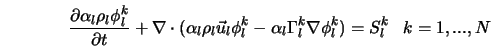# Write UDS parameters

Hi, community,

I saw that there is the “scalar” option to add a new scalar transport equation. My aim is to write the terms of diffusion and addition of this “scalar”.In the documentation, there is the file `scalar_prop.f` for “Properties and source terms in scalar transport equations”.

1. Is this a Eulerian or Lagrangian derivative?

scalar_prop.f:55: ! d (Scalar)/dt = S

1. How can I do the second stage? I think I should call the variable “Scalar” (IJK, L) from some other subroutine again, but how can I do that?

S^{k1}_g = - S^{k2}_m = Scalar_c - Scalar_p * S^{k1}_g
S^{k1}_g = - S^{k2}_m

Best Regards,

1 Like